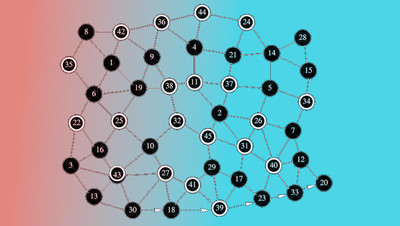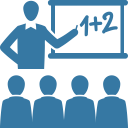# Discrete Mathematics

Educator Edition

 Course Discrete Mathematics: Logic, mathematical reasoning, introduction to proofs, mathematical induction, set operations, algorithms, introduction to number theory, recurrence relations, techniques of counting, graphs, as well as specific questions given by the “Towers of Hanoi,” and Euler’s “7 bridges of Konigsberg problem.” Textbook This course is frequently paired with the following textbook(s): Discrete Mathematics with Applications (7th edition or earlier) by Kenneth Rosen

Edfinity is a full-featured homework system that supports mathematically aware problems with algebraic input, evaluation of mathematical expressions, and randomized variants for personalized learning. Use this problem series as-is, or mix-and-match with other series or your own problems as desired. Edfinity gives you complete control over the every aspect of students' online assessment experience.

## How to use this course

• Develop problem-solving skills: Use the problem sets to help students develop problem-solving skills. Save hours of grading time.
• Flip your classroom: Have students work on assignments ahead of class to master the basics and save precious class time for discussions and difficult problems.
• Make your own tests and quizzes: Edfinity’s flexible assignment templates allow you to create timed quizzes and tests in minutes. Assessments are auto-scored and provide summary statistics and analytics on student outcomes
• Remix problems: Select problem sets of your choice. Find more problems in the Edfinity repository, or create your own.

## WeBWorK

This course includes algorithmic WeBWorK problems, with support for randomized variants, algebraic expressions, and mathematical objects such as vectors, matrices, intervals, and complex numbers.

## Problem Sets

1. Edfinity Demo
2. 1.6 Rules of Inference; 1.7 Introduction to Proofs
3. 1.8 Methods of Proof and Strategy; 2.1 Sets
4. 2.2 Set Operations; 2.3 Functions; 2.4 Sequences and Summations
5. 3.1 Algorithms; 3.2 Growth of Functions
6. 3.3 Complexity of Algorithms; 4.1 Divisibility and Modular Arithmetic
7. 4.4 Solving Congruences; 4.5 Applications of Congruences
8. 4.6 Cryptography; 5.1 Mathematical Induction
9. 5.4 Recursive Algorithms; 6.1 Basics of Counting; 6.2 Pigeonhole Principle
10. 8.2 Solving Linear Recurrence Relations; 10.1 Graph and Graph Model; 10.2 Graph Terminology and Special Types of Graphs
11. 10.3 Representing Graphs and Graph Isomorphism; 10.4 Connectivity; 10.5 Euler and Hamilton Paths and Circuits

##License

This is a multi-student, educator license. You will be able to manage an online course with your students, and monitor their progress.

Price: FREE
Trusted by## Try problems from this course

Cookies help us deliver our services. By using our services, you agree to our use of cookies.